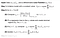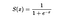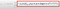# Gradient Boosting

Wow, I am glad I finally understood this ❤.

Starting from our understanding of AdaBoost.

Adaboost

1. Assume for Regression
2. First of all build a small tree from training data called stump
3. Using the errors as weight for that observation(or use that observation weight number of times), build another stump.
4. Repeat step 3, to required number of iterations.

Now Gradient Boosting operates in same manner, with difference coming

• Where instead of small stump, GBM builds a full tree(often restricted to depth). Calculate error= Predicted value - Actual Value. Note predicted value in first iteration is average value of all target variables( i.e. we start with a single node tree)
• Later instead of using errors as weights, these errors are taken as target variable to construct a new decision tree(such that all similar value errors are grouped together).
• Post completing this, we arrive at a average error for each leaf.
• Following each path of decision tree, we predict the actual target variable by adding this error to our prediction at first step(same as average value in first iteration).
• Note simply adding average error to predicted value would lead to overfitting. So we instead attach a weight called learning rate to our error term. So in total it becomes New prediction = Old Prediction + learning rate* average error.
• We repeat all above steps to number of iterations.
• It is common to get different trees at each step while constructing trees for errors, but that’s fine. Errors in each iteration would reduce after adding new tree of errors, showing we are slowing moving towards our most wanted prediction.

All of the above can be seen mathematically as belowMAIN ALGORITHM

The final Output F_{M}(x) is the required prediction that we reached to in M iteration post building M trees of residuals.

The above steps can be easily seen if we plug loss function to be

1/2(Predicted — Actual)². This can be seen to calculate F0 to be average of all observations. And gamma(jm) to be average of residuals in leaf Jm.

This same thing can be replicated for Classification Problem as well. The only thing changes is the loss function which is Log(Odds) most commonly.

GBM operates in similar manner with changes

• Start with F0 as log(odds) say log(#1/#0) where #1: No. of observations with label 1.
• Next transform this into probability by sigmoid function. i.e.x = log(odds)
• Find residuals like 1-S(log(odds)) and 0-S(log(odds)) for observations with 1 and 0 class labels respectively.
• Build regression tree for these residuals.
• Unlike Regression GBM(i.e. averaging residuals in each leaf), we need some transformation to these residuals before adding them into our previous prediction(log odds probability in first iteration). Most common one is
• We calculate above expression for each leaf of residual regression tree. Note in first iteration previous probability would be same as S(F0). But it would be different for each observation in next iterations.
• New predictions are now calculated using previous log odds+ learning rate times above expression based on the leaf (of regression tree) in which that observation falls.
• Before moving on to next iteration we convert above into a probability by using sigmoid function again.
• This final probability for a given observation is the starting probability F1 for that observation.
• Repeat the steps for specified iterations.MAIN ALGORITHM

This can be again seen from Main Algorithm by substituting for a loss function to be log likelihood, and differentiating to arrive at probabilities.

Note, this can be tweaked to arrive at

Initial Prediction comes from differentiating this function with respect to log(odds) and finding the log odds which minimizes this loss function. It comes out F0 to be log(#1/#0) as taken earlier.

In next step, we calculate residuals, and then construct regression trees with these residuals as target variable.

We arrive at step © in above algorithm, where we need to find gamma(jm) that is score for each leaf. Note after pulling in the loss fuction with parameters as in ©, we getfor first leaf, m-1 tree

We need to find score that minimizes Loss function at that leaf. We could differentiate this, but it would be messy so we instead use Gradient Boost.Second order Taylor Approximation of polynomial

Instead of taking entire polynomial we use its Taylor expansion, and then take derivative w.r.t. gamma. which gives us

After setting this expression to 0, as we do to find point of minima, this gives us

Second derivative in denominator comes out to

This result is same as we used earlier

So we perform next iteration to get F1 prediction,

Using the sigmoid function, we get probability of 1, if S(FM)>0.5 and 0 otherwise.

# MY SOCIAL SPACES

Problem Solver, Data Science, Actuarial Science, Knowledge Sharer, Hardcore Googler

## More from Shaily jain

Problem Solver, Data Science, Actuarial Science, Knowledge Sharer, Hardcore Googler